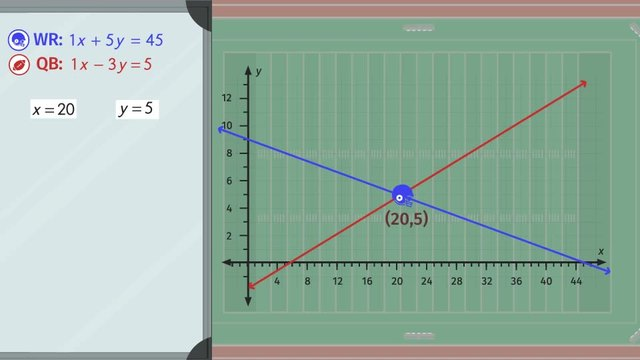# The Elimination MethodRating

Be the first to give a rating!
The authorsEugene Lee

## Basics on the topicThe Elimination Method

After this lesson, you will be able to solve systems of equations by elimination.

The lesson begins by teaching you that the goal of this method is to eliminate one of the variables first. It leads you to learn how to change or manipulate the equations before you can add them together to eliminate that variable. It concludes by showing you how to then solve for the eliminated variable to get the solution.

Learn about the elimination method by watching the 79ers practice for their football game.

This video includes key concepts, notation, and vocabulary such as whole number constant, which is the number we multiply an equation by to change or manipulate it to get the additive inverse required to eliminate a variable.

Before watching this video, you should already be familiar with the distributive property, additive inverses, the least common multiple, and opposite operations.

After watching this video, you will be prepared to identify real-world situations that may require finding a common point of intersection between two equations and solve them using elimination.

Common Core Standard(s) in focus: 8.EE.C.8 A video intended for math students in the 8th grade Recommended for students who are 13 - 14 years old

### TranscriptThe Elimination Method

Last Friday, the 79ers football team lost really badly, so to avoid being embarrassed again, some of the players decide to stay after practice. The coach has given each of the players a linear equation to determine where they should be on the field. In order to be more successful in the next game and for the rest of the season, the players need to know how to solve linear systems of equations using the elimination method. The coach’s odd playbook calls for his wide receiver to run according to the linear equation ‘x’ plus 5y equals 45. Montana Jo, the star quarterback, needs to throw the ball with the linear equation 'x' minus 3y equals 5. But where will the ball and the receiver meet? Will they meet? Let’s help the receiver figure out where he’ll be able to catch the ball. To make things easier to see, let’s put a cartesian coordinate system over the football field. When using the elimination method on a system of linear equations, the goal is to ELIMINATE one of the variables, but there are some rules you’ll need to follow. If you notice, the coefficient for the variable ‘x’ in each linear equation is positive 1. However, the coefficients for the variable ‘y’ are 5 and negative 3, respectively. Here, we can use the elimination method for solving two linear equations. The goal is to manipulate, or change, an equation so that when we add the two equations together, we eliminate one of the variables. If we multiply the second equation by negative 1, we distribute to get negative ‘x’ plus 3y equals negative 5. "Now, if we add our two linear equations together, we’ll be able to eliminate the ‘x’s, giving us 0x plus 8y equals 40. Now that we’ve gotten rid of the variable ‘x’ in our equations, we can simply solve for ‘y’ using opposite operations."